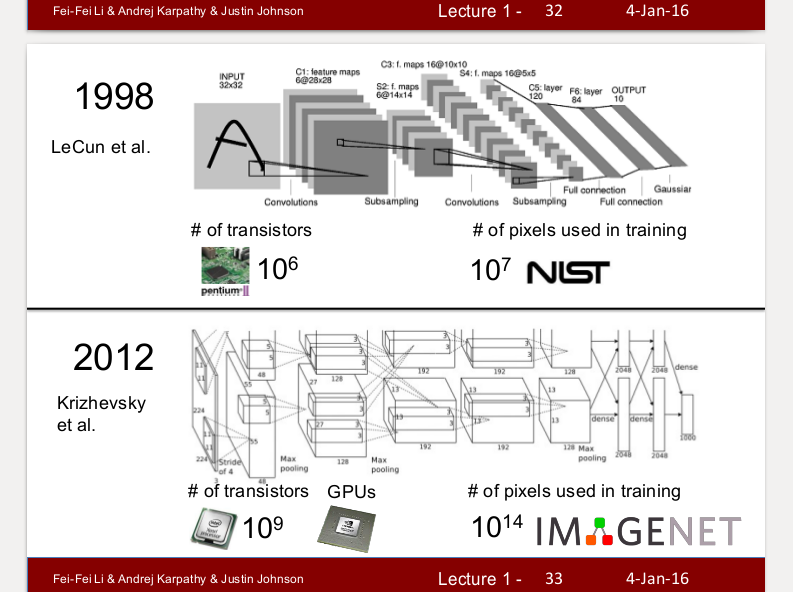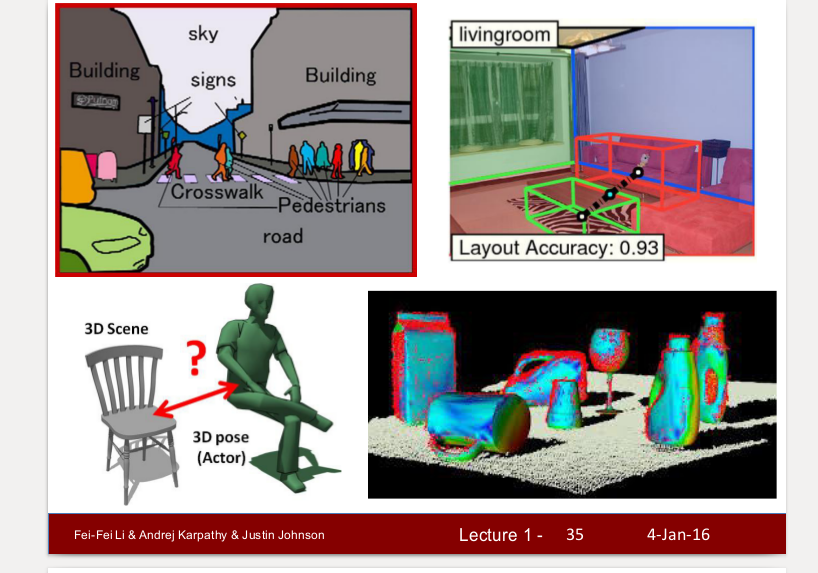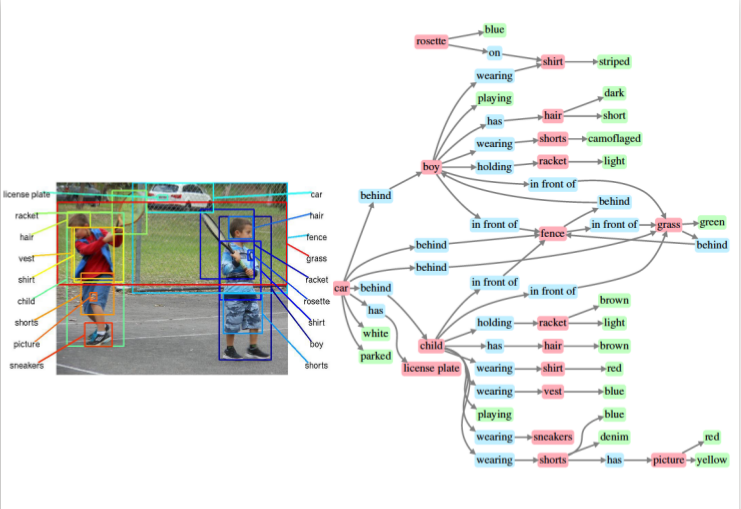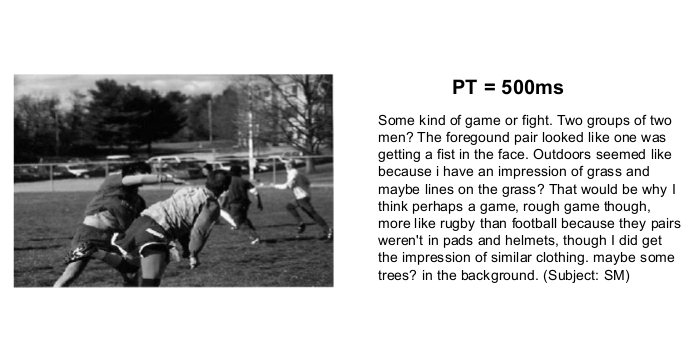2018-07-13 16:21:39 weixin_38267719 阅读数 1148

之前为了熟悉机器学习的东西去搞kaggle的东西，然后就从Titanic入门咯，结果发现并没有对机器学习的东西有深入的理解，做数据挖掘的时候直接调用sklearn里面的框架，根本不用去想机器学习的公式的运用和基础的实现，想用SVM就直接from sklearn import svm，然后clf = svm.SVC(kernel='linear', C=1)往这里面填几个参数，知道基本原理和参数的含义就行。直到我看到这段话：

https://zhuanlan.zhihu.com/p/21930884     大牛搞的CS231n官方笔记授权翻译总集

http://cs231n.stanford.edu/2016/syllabus    英文的CS231n官方笔记

http://www.cs.toronto.edu/~kriz/cifar.html    做学习的数据集

## 一、概念&意义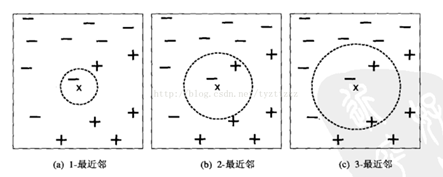## 二、计算步骤：

1算距离：给定测试对象，计算它与训练对象距离

2找邻居：圈定距离最近的 k个对象，作为测试对象的近邻

3做分类：根据这k个近邻归属的主要类别，来对测试对象分类

## 三、三个基本要素

### I、距离度量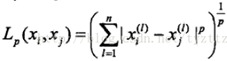a)p=2时，为欧式距离：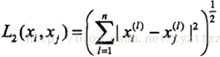b)p=1时，为曼哈顿距离：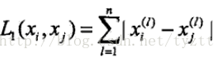### II、K值的选择

K值越小，整体模型越复杂，容易发生过拟合

K值越大，整体模型越简单，近似误差会增大（误分类）

### III、测试对象类别的判定

a)   多数表决：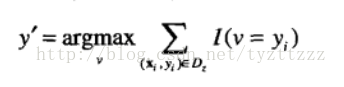b)   距离加权表决：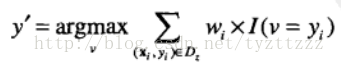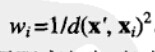# 损失函数的公式L = -(1/N)∑i∑j1(k=yi)log(exp(fk)/∑j exp(fj)) +λR(W)

import numpy as np
class KNearestNeighbor(object):#首先是定义一个处理KNN的类
""" a kNN classifier with L2 distance """

def __init__(self):
pass

def train(self, X, y):
self.X_train = X
self.y_train = y

def predict(self, X, k=1, num_loops=0):
if num_loops == 0:
dists = self.compute_distances_no_loops(X)
elif num_loops == 1:
dists = self.compute_distances_one_loop(X)
elif num_loops == 2:
dists = self.compute_distances_two_loops(X)
else:
raise ValueError('Invalid value %d for num_loops' % num_loops)

return self.predict_labels(dists, k=k)

def compute_distances_two_loops(self, X):
num_test = X.shape
num_train = self.X_train.shape
dists = np.zeros((num_test, num_train))
for i in xrange(num_test):
for j in xrange(num_train):
dists[i][j] = np.sqrt(np.sum(np.square(self.X_train[j,:] - X[i,:])))
return dists

def compute_distances_one_loop(self, X):
num_test = X.shape
num_train = self.X_train.shape
dists = np.zeros((num_test, num_train))
for i in range(num_test):   #i就是类别，i=1即类1
dists[i,:] = np.sqrt(np.sum(np.square(self.X_train-X[i,:]),axis = 1))
return dists

def compute_distances_no_loops(self, X):
num_test = X.shape
num_train = self.X_train.shape
dists = np.zeros((num_test, num_train))   #dists(测试类别数，测试集的数量)
dists = np.multiply(np.dot(X,self.X_train.T),-2)
sq1 = np.sum(np.square(X),axis=1,keepdims = True)
sq2 = np.sum(np.square(self.X_train),axis=1)
dists = np.sqrt(dists)
return dists

def predict_labels(self, dists, k=1):
num_test = dists.shape
y_pred = np.zeros(num_test)
for i in range(num_test):
closest_y = []
closest_y = self.y_train[np.argsort(dists[i,:])[:k]]
y_pred[i]=np.argmax(np.bincount(closest_y))
return y_pred

python自己基本上算是没学过，靠着C++的底子强行直接上了，给自己留一点提示吧
def __init__(self):
pass   #一般不pass，一般def __init__(self,X,y):self.X=X，就是把外部传入的变量变成类内部的东西，当然也可以像代码中再搞个方法来做这个步骤
def train(self, X, y):
self.X_train = X
self.y_train = y

if num_loops == 0:
dists = self.compute_distances_no_loops(X)
elif num_loops == 1:
dists = self.compute_distances_one_loop(X)
elif num_loops == 2:
dists = self.compute_distances_two_loops(X)

np.argsort(dists[i,:])对每一种num_test_picture对应的num_train_picture个距离进行排序
closest_y = self.y_train[np.argsort(dists[i,:])[:k]]  然后对每一种num_test_picture取前k个到closest_y，closest_y
p.argmax:最大值的索引
x = np.array([0, 1, 1, 3, 2, 1, 7])  索引0出现了1次，索引1出现了3次......索引5出现了0次...... np.bincount(x)，因此，输出结果为：array([1, 3,1, 1, 0, 0, 0, 1])
y_pred[i] = np.argmax(np.bincount(closest_y))#就把索引出现最多的那个所以赋值到y_pred[i]

# -*- coding: utf-8 -*-
import pickle as p
import numpy as np
import os
import KNearestNeighbor
""" 载入cifar数据集的一个batch """
with open(filename, 'rb') as f:
X = X.reshape(10000, 3, 32, 32).transpose(0, 2, 3, 1).astype("float")
Y = np.array(Y)
return X, Y

""" 载入cifar全部数据 """
xs = []
ys = []
for b in range(1, 2):
f = os.path.join(ROOT, 'data_batch_%d' % (b,))
xs.append(X)         #将所有batch整合起来
ys.append(Y)
Xtr = np.concatenate(xs) #使变成行向量,最终Xtr的尺寸为(50000,32*32*3)
Ytr = np.concatenate(ys)
del X, Y
return Xtr, Ytr, Xte, Yte

import numpy as np
import matplotlib.pyplot as plt
plt.rcParams['figure.figsize'] = (10.0, 8.0)
plt.rcParams['image.interpolation'] = 'nearest'
plt.rcParams['image.cmap'] = 'gray'

# 载入CIFAR-10数据集
cifar10_dir = 'julyedu/datasets/cifar-10-batches-py'
X_train, y_train, X_test, y_test = load_CIFAR10(cifar10_dir)

X_train=np.reshape(X_train,(X_train.shape,-1))
X_test=np.reshape(X_test,(X_test.shape,-1))

X_train=X_train[0:10000,:]
X_test=X_test[0:500,:]
y_train=y_train[0:10000]
y_test=y_test[0:500]

for k in [1, 3, 5, 10, 20, 50, 100]:
# use a particular value of k and evaluation on validation data
nn = KNearestNeighbor.KNearestNeighbor() #这里有两个KNearestNeighbor，一个是文件，后面的是文件里的类
nn.train(X_train, y_train)
# here we assume a modified NearestNeighbor class that can take a k as input
Yval_predict = nn.predict(X_test, k = k)
acc = np.mean(Yval_predict == y_test)
print( 'accuracy: %f' % (acc,))

accuracy: 0.276000
accuracy: 0.304000
accuracy: 0.324000
accuracy: 0.306000
accuracy: 0.298000
accuracy: 0.264000
accuracy: 0.262000

2018-01-20 00:00:00 dQCFKyQDXYm3F8rB0 阅读数 5698CMU: 深度学习

Yoshua Bengio: 深度学习

UC Berkeley: 深度强化学习

Yoshua Bengio: 深度学习与强化学习暑期学校

CMU: 深度强化学习与控制

CMU: 深度学习入门

RLDM: 深度强化学习入门

UC Berkeley: 深度强化学习入门

MLSS: 深度强化学习入门

AI校招程序员最高薪酬曝光！腾讯80万年薪领跑，还送北京户口2018-05-29 14:37:54 jichihui7464 阅读数 1982

k近邻分类器工作方式是高效的得到本联合训练集。

python处理数据基础：

``````import numpy as np

# Create the following rank 2 array with shape (3, 4)
# [[ 1  2  3  4]
#  [ 5  6  7  8]
#  [ 9 10 11 12]]
a = np.array([[1,2,3,4], [5,6,7,8], [9,10,11,12]])

# Two ways of accessing the data in the middle row of the array.
# Mixing integer indexing with slices yields an array of lower rank,
# while using only slices yields an array of the same rank as the
# original array:
row_r1 = a[1, :]    # Rank 1 view of the second row of a
row_r2 = a[1:2, :]  # Rank 2 view of the second row of a
print(row_r1, row_r1.shape)  # Prints "[5 6 7 8] (4,)"
print(row_r2, row_r2.shape)  # Prints "[[5 6 7 8]] (1, 4)"

# We can make the same distinction when accessing columns of an array:
col_r1 = a[:, 1]
col_r2 = a[:, 1:2]
print(col_r1, col_r1.shape)  # Prints "[ 2  6 10] (3,)"
print(col_r2, col_r2.shape)  # Prints "[[ 2]
#          [ 6]
#          ] (3, 1)"``````

python中处理数组与matlab是不一样的。

When you index into numpy arrays using slicing, the resulting array view will always be a subarray of the original array. In contrast, integer array indexing allows you to construct arbitrary arrays using the data from another array. Here is an example：

``````import numpy as np

a = np.array([[1,2], [3, 4], [5, 6]])

# An example of integer array indexing.
# The returned array will have shape (3,) and
print(a[[0, 1, 2], [0, 1, 0]])  # Prints "[1 4 5]"

# The above example of integer array indexing is equivalent to this:
print(np.array([a[0, 0], a[1, 1], a[2, 0]]))  # Prints "[1 4 5]"``````

bool值应用于数组中选取符合条件的数据。

``````import numpy as np

a = np.array([[1,2], [3, 4], [5, 6]])

bool_idx = (a > 2)   # Find the elements of a that are bigger than 2;
# this returns a numpy array of Booleans of the same
# shape as a, where each slot of bool_idx tells
# whether that element of a is > 2.

print(bool_idx)      # Prints "[[False False]
#          [ True  True]
#          [ True  True]]"

# We use boolean array indexing to construct a rank 1 array
# consisting of the elements of a corresponding to the True values
# of bool_idx
print(a[bool_idx])  # Prints "[3 4 5 6]"

# We can do all of the above in a single concise statement:
print(a[a > 2])     # Prints "[3 4 5 6]"``````

numpy与matlab进行点乘与数乘时的不同之处：

Note that unlike MATLAB, `*` is elementwise multiplication, not matrix multiplication. We instead use the `dot`function to compute inner products of vectors, to multiply a vector by a matrix, and to multiply matrices. `dot` is available both as a function in the numpy module and as an instance method of array objects:

``````import numpy as np

x = np.array([[1,2],[3,4]])
y = np.array([[5,6],[7,8]])

v = np.array([9,10])
w = np.array([11, 12])

# Inner product of vectors; both produce 219
print(v.dot(w))
print(np.dot(v, w))

# Matrix / vector product; both produce the rank 1 array [29 67]
print(x.dot(v))
print(np.dot(x, v))

# Matrix / matrix product; both produce the rank 2 array
# [[19 22]
#  [43 50]]
print(x.dot(y))
print(np.dot(x, y))``````

Numpy provides many useful functions for performing computations on arrays; one of the most useful is `sum`:

numpy提供的矩阵加法，分为行加，列加或全加。

``````import numpy as np

x = np.array([[1,2],[3,4]])

print(np.sum(x))  # Compute sum of all elements; prints "10"
print(np.sum(x, axis=0))  # Compute sum of each column; prints "[4 6]"
print(np.sum(x, axis=1))  # Compute sum of each row; prints "[3 7]"``````

numpy中提供的转置的方法：

Apart from computing mathematical functions using arrays, we frequently need to reshape or otherwise manipulate data in arrays. The simplest example of this type of operation is transposing a matrix; to transpose a matrix, simply use the `T` attribute of an array object:

``````import numpy as np

x = np.array([[1,2], [3,4]])
print(x)    # Prints "[[1 2]
#          [3 4]]"
print(x.T)  # Prints "[[1 3]
#          [2 4]]"

# Note that taking the transpose of a rank 1 array does nothing:
v = np.array([1,2,3])
print(v)    # Prints "[1 2 3]"
print(v.T)  # Prints "[1 2 3]"``````

Broadcasting is a powerful mechanism that allows numpy to work with arrays of different shapes when performing arithmetic operations. Frequently we have a smaller array and a larger array, and we want to use the smaller array multiple times to perform some operation on the larger array.

``````import numpy as np

# We will add the vector v to each row of the matrix x,
# storing the result in the matrix y
x = np.array([[1,2,3], [4,5,6], [7,8,9], [10, 11, 12]])
v = np.array([1, 0, 1])
y = x + v  # Add v to each row of x using broadcasting
print(y)  # Prints "[[ 2  2  4]
#          [ 5  5  7]
#          [ 8  8 10]
#          [11 11 13]]"``````

2018-01-23 20:59:57 PRIMEFJT 阅读数 688### MLSS: 深度强化学习入门

2019-07-23 22:35:56 Arthur_Holmes 阅读数 136

https://www.bilibili.com/video/av18443065/?p=4### 85%的数据都是图像视频数据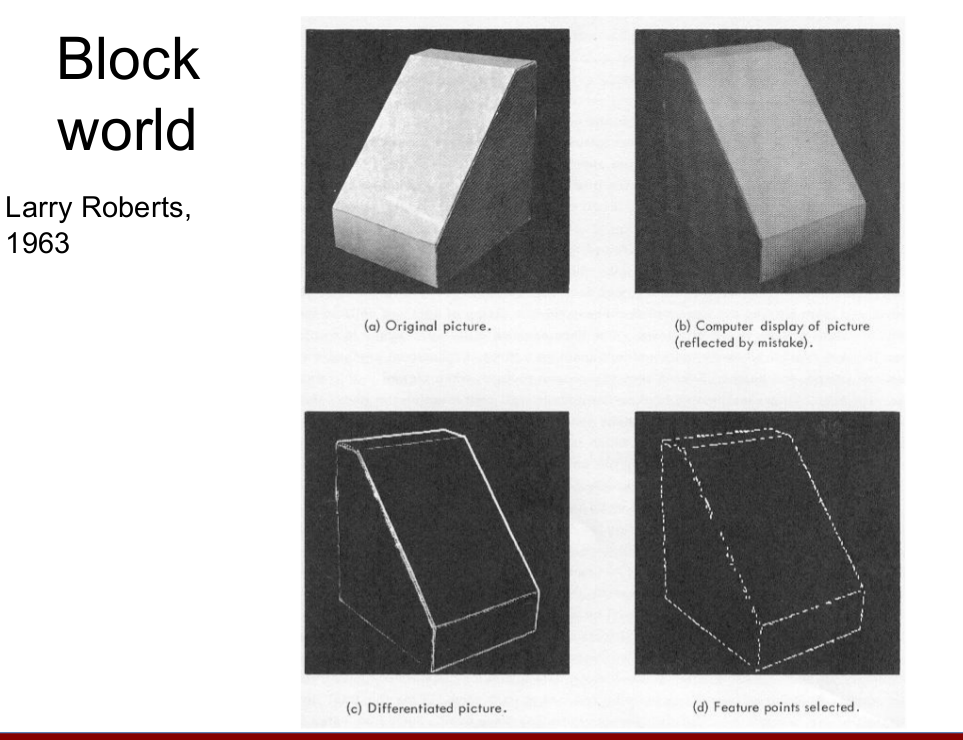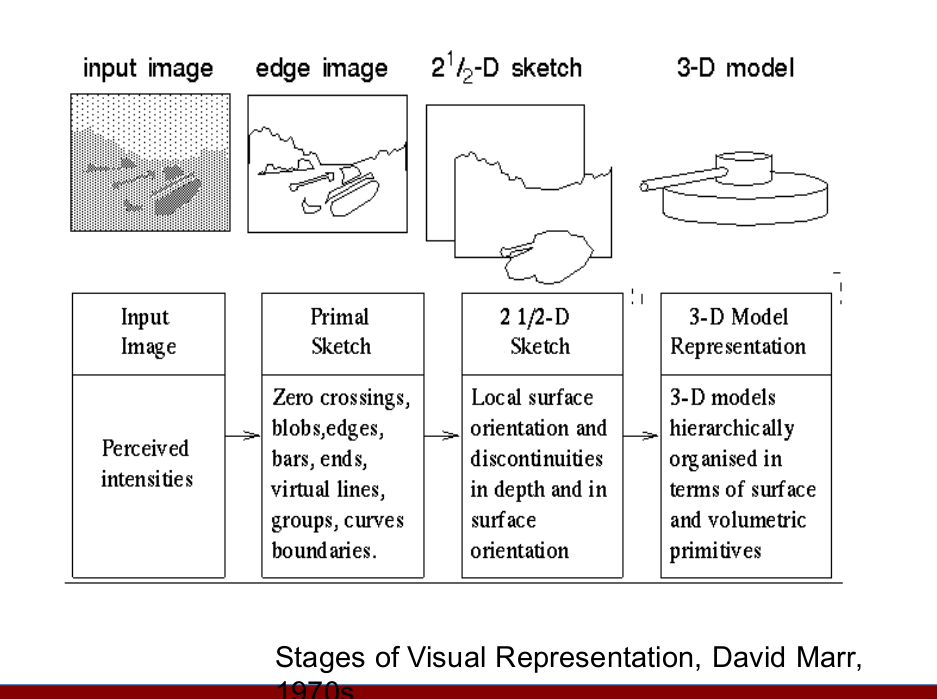### 从三维建模跳到了识别，回到了AI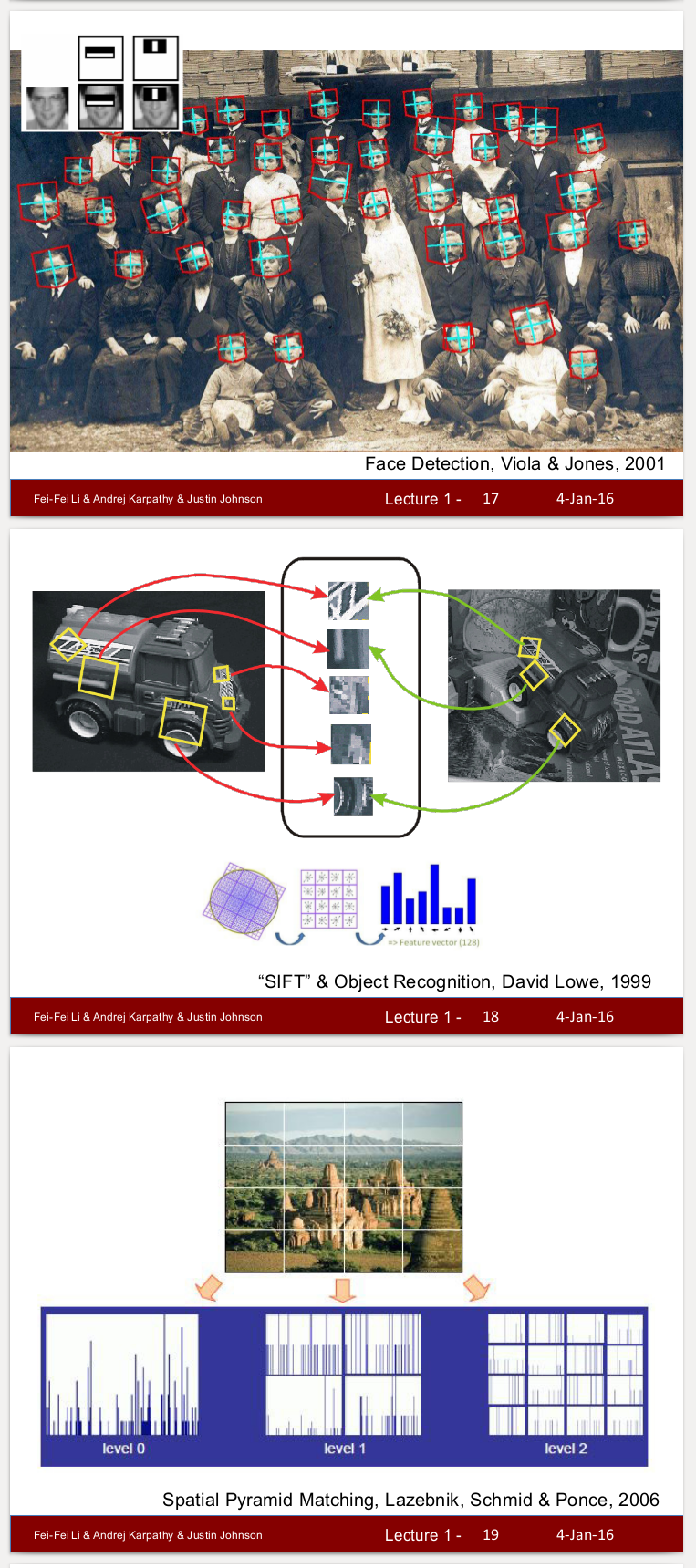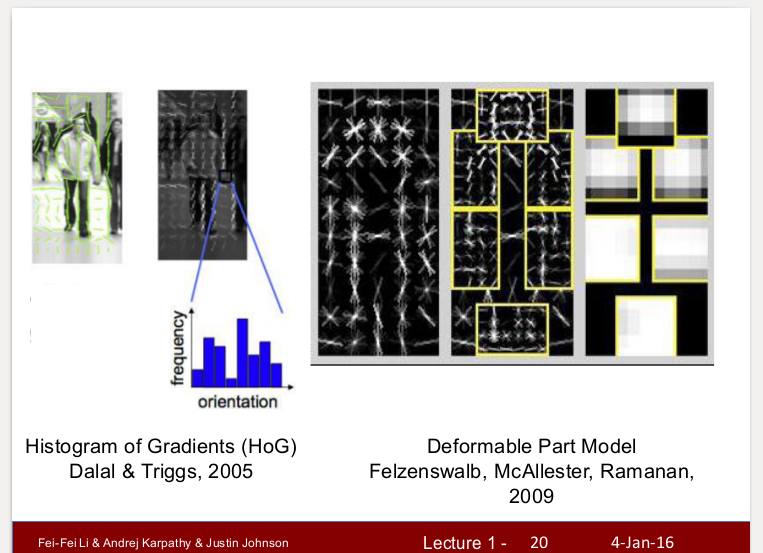### 2012CNN模型使用